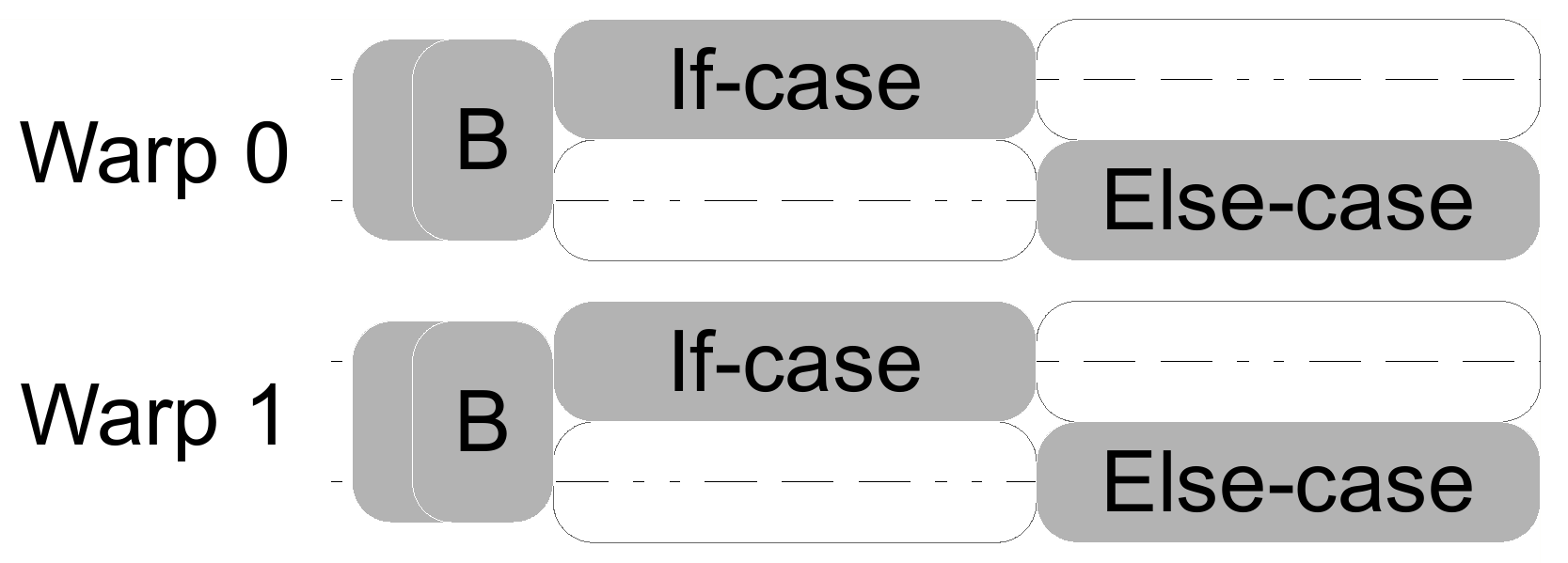# GPU branch divergence

This pattern applies to GPU computing, where the execution of a thread block is divided into warps with a constant number of threads per warp. When threads in the same warp follow different paths of control flow, these threads diverge in their execution, which serializes the execution.

Branch divergence can hurt performance as it lowers utilization of the execution units, which cannot be compensated for through increased levels of parallelism.

There are three common scenarios of kernel code that exhibit such divergence:

// Code A
if(a[tid] > 0) {
++x;
}


In this scenario, a single if statement, if any thread executes ++x, all threads in the same warp must go through ++x, regardless of whether they actually execute it or not.

The more common case, as depicted in the figure above is the if-else statement:

// Code B
if(a[tid] > 0) {
++x;
} else {
--x;
}


Each thread in a warp must go through both branch paths sequentially, even though it just executes one of them. Of course, this scenario could be expanded with one or more else if statements (equivalent to a switch statement), which would add further complexity to the issue.

Last but not least, in the following code snippet, both branches perform the same operations on different data:

// Code C
if(c > 0) {
x = x * a1 + b1;
y = y * a1 + b1;
} else {
x = x * a2 + b2;
y = y * a2 + b2;
}


The branch divergence pattern is quite evident in the first three scenarios. However, there is a fourth scenario, which is a bit more tricky.

// Code D

Here we have a loop with a variable trip count. The number of iterations each thread goes through is the max iteration count i of all threads within the warp. The performance impact depends on the size of the loop body and the variance of the loop trip counts, i.e., the n’s.